# Soft Actor-Critic (SAC)

## Overview

The Soft Actor-Critic (SAC) algorithm extends the DDPG algorithm by 1) using a stochastic policy, which in theory can express multi-modal optimal policies. This also enables the use of 2) entropy regularization based on the stochastic policy's entropy. It serves as a built-in, state-dependent exploration heuristic for the agent, instead of relying on non-correlated noise processes as in DDPG, or TD3 Additionally, it incorporates the 3) usage of two Soft Q-network to reduce the overestimation bias issue in Q-network-based methods.

Original papers: The SAC algorithm's initial proposal, and later updates and improvements can be chronologically traced through the following publications:

Reference resources:

Variants Implemented Description
sac_continuous_actions.py, docs For continuous action spaces
sac_atari.py, docs For discrete action spaces

Below are our single-file implementations of SAC:

## sac_continuous_action.py

The sac_continuous_action.py has the following features:

• For continuous action spaces.
• Works with the Box observation space of low-level features.
• Works with the Box (continuous) action space.
• Numerically stable stochastic policy based on openai/spinningup and pranz24/pytorch-soft-actor-critic implementations.
• Supports automatic entropy coefficient $$\alpha$$ tuning, enabled by default.

### Usage for continuous action spaces

poetry install
poetry install -E mujoco
poetry run python cleanrl/sac_continuous_action.py --help
poetry run python cleanrl/sac_continuous_action.py --env-id Hopper-v4
poetry install -E mujoco_py # only works in Linux
poetry run python cleanrl/sac_continuous_action.py --env-id Hopper-v2
poetry run python cleanrl/sac_continuous_action.py --env-id Hopper-v2 --autotune False --alpha 0.2 ## Without Automatic entropy coef. tuning

pip install -r requirements/requirements-mujoco.txt
python cleanrl/sac_continuous_action.py --help
python cleanrl/sac_continuous_action.py --env-id Mujoco-v4
pip install -r requirements/requirements-mujoco_py.txt # only works in Linux
python cleanrl/sac_continuous_action.py --env-id Hopper-v2
python cleanrl/sac_continuous_action.py --env-id Hopper-v2 --autotune False --alpha 0.2 ## Without Automatic entropy coef. tuning


### Explanation of the logged metrics

Running python cleanrl/sac_continuous_action.py will automatically record various metrics such as actor or value losses in Tensorboard. Below is the documentation for these metrics:

• charts/episodic_return: the episodic return of the game during training

• charts/SPS: number of steps per second

• losses/qf1_loss, losses/qf2_loss: for each Soft Q-value network $$Q_{\theta_i}$$, $$i \in \{1,2\}$$, this metric holds the mean squared error (MSE) between the soft Q-value estimate $$Q_{\theta_i}(s, a)$$ and the entropy regularized Bellman update target estimated as $$r_t + \gamma \, Q_{\theta_{i}^{'}}(s', a') + \alpha \, \mathcal{H} \big[ \pi(a' \vert s') \big]$$.

More formally, the Soft Q-value loss for the $$i$$-th network is obtained by:

$J(\theta^{Q}_{i}) = \mathbb{E}_{(s,a,r,s') \sim \mathcal{D}} \big[ (Q_{\theta_i}(s, a) - y)^2 \big]$

with the entropy regularized, Soft Bellman update target: $$y = r(s, a) + \gamma ({\color{orange} \min_{\theta_{1,2}}Q_{\theta_i^{'}}(s',a')} - \alpha \, \text{log} \pi( \cdot \vert s'))$$ where $$a' \sim \pi( \cdot \vert s')$$, $$\text{log} \pi( \cdot \vert s')$$ approximates the entropy of the policy, and $$\mathcal{D}$$ is the replay buffer storing samples of the agent during training.

Here, $$\min_{\theta_{1,2}}Q_{\theta_i^{'}}(s',a')$$ takes the minimum Soft Q-value network estimate between the two target Q-value networks $$Q_{\theta_1^{'}}$$ and $$Q_{\theta_2^{'}}$$ for the next state and action pair, so as to reduce over-estimation bias.

• losses/qf_loss: averages losses/qf1_loss and losses/qf2_loss for comparison with algorithms using a single Q-value network.

• losses/actor_loss: Given the stochastic nature of the policy in SAC, the actor (or policy) objective is formulated so as to maximize the likelihood of actions $$a \sim \pi( \cdot \vert s)$$ that would result in high Q-value estimate $$Q(s, a)$$. Additionally, the policy objective encourages the policy to maintain its entropy high enough to help explore, discover, and capture multi-modal optimal policies.

The policy's objective function can thus be defined as:

$\text{max}_{\phi} \, J_{\pi}(\phi) = \mathbb{E}_{s \sim \mathcal{D}} \Big[ \text{min}_{i=1,2} Q_{\theta_i}(s, a) - \alpha \, \text{log}\pi_{\phi}(a \vert s) \Big]$

where the action is sampled using the reparameterization trick1: $$a = \mu_{\phi}(s) + \epsilon \, \sigma_{\phi}(s)$$ with $$\epsilon \sim \mathcal{N}(0, 1)$$, $$\text{log} \pi_{\phi}( \cdot \vert s')$$ approximates the entropy of the policy, and $$\mathcal{D}$$ is the replay buffer storing samples of the agent during training.

• losses/alpha: $$\alpha$$ coefficient for entropy regularization of the policy.

• losses/alpha_loss: In the policy's objective defined above, the coefficient of the entropy bonus $$\alpha$$ is kept fixed all across the training. As suggested by the authors in Section 5 of the Soft Actor-Critic And Applications paper, the original purpose of augmenting the standard reward with the entropy of the policy is to encourage exploration of not well enough explored states (thus high entropy). Conversely, for states where the policy has already learned a near-optimal policy, it would be preferable to reduce the entropy bonus of the policy, so that it does not become sub-optimal due to the entropy maximization incentive.

Therefore, having a fixed value for $$\alpha$$ does not fit this desideratum of matching the entropy bonus with the knowledge of the policy at an arbitrary state during its training.

To mitigate this, the authors proposed a method to dynamically adjust $$\alpha$$ as the policy is trained, which is as follows:

$\alpha^{*}_t = \text{argmin}_{\alpha_t} \mathbb{E}_{a_t \sim \pi^{*}_t} \big[ -\alpha_t \, \text{log}\pi^{*}_t(a_t \vert s_t; \alpha_t) - \alpha_t \mathcal{H} \big],$

where $$\mathcal{H}$$ represents the target entropy, the desired lower bound for the expected entropy of the policy over the trajectory distribution induced by the latter. As a heuristic for the target entropy, the authors use the dimension of the action space of the task.

### Implementation details

CleanRL's sac_continuous_action.py implementation is based on openai/spinningup.

1. sac_continuous_action.py uses a numerically stable estimation method for the standard deviation $$\sigma$$ of the policy, which squashes it into a range of reasonable values for a standard deviation:

LOG_STD_MAX = 2
LOG_STD_MIN = -5

class Actor(nn.Module):
def __init__(self, env):
super(Actor, self).__init__()
self.fc1 = nn.Linear(np.array(env.single_observation_space.shape).prod(), 256)
self.fc2 = nn.Linear(256, 256)
self.fc_mean = nn.Linear(256, np.prod(env.single_action_space.shape))
self.fc_logstd = nn.Linear(256, np.prod(env.single_action_space.shape))
# action rescaling
self.action_scale = torch.FloatTensor((env.action_space.high - env.action_space.low) / 2.0)
self.action_bias = torch.FloatTensor((env.action_space.high + env.action_space.low) / 2.0)

def forward(self, x):
x = F.relu(self.fc1(x))
x = F.relu(self.fc2(x))
mean = self.fc_mean(x)
log_std = self.fc_logstd(x)
log_std = torch.tanh(log_std)
log_std = LOG_STD_MIN + 0.5 * (LOG_STD_MAX - LOG_STD_MIN) * (log_std + 1)  # From SpinUp / Denis Yarats

return mean, log_std

def get_action(self, x):
mean, log_std = self(x)
std = log_std.exp()
normal = torch.distributions.Normal(mean, std)
x_t = normal.rsample()  # for reparameterization trick (mean + std * N(0,1))
y_t = torch.tanh(x_t)
action = y_t * self.action_scale + self.action_bias
log_prob = normal.log_prob(x_t)
# Enforcing Action Bound
log_prob -= torch.log(self.action_scale * (1 - y_t.pow(2)) + 1e-6)
log_prob = log_prob.sum(1, keepdim=True)
mean = torch.tanh(mean) * self.action_scale + self.action_bias
return action, log_prob, mean

def to(self, device):
self.action_scale = self.action_scale.to(device)
self.action_bias = self.action_bias.to(device)
return super(Actor, self).to(device)


Note that unlike openai/spinningup's implementation which uses LOG_STD_MIN = -20, CleanRL's uses LOG_STD_MIN = -5 instead.

2. sac_continuous_action.py uses different learning rates for the policy and the Soft Q-value networks optimization.

    parser.add_argument("--policy-lr", type=float, default=3e-4,
help="the learning rate of the policy network optimizer")
help="the learning rate of the Q network network optimizer")


while openai/spinningup's uses a single learning rate of lr=1e-3 for both components.

Note that in case it is used, the automatic entropy coefficient $$\alpha$$'s tuning shares the q-lr learning rate:

    # Automatic entropy tuning
if args.autotune:
target_entropy = -torch.prod(torch.Tensor(envs.single_action_space.shape).to(device)).item()
alpha = log_alpha.exp().item()
else:
alpha = args.alpha

3. sac_continuous_action.py uses --batch-size=256 while openai/spinningup's uses --batch-size=100 by default.

### Pybullet experiment results for SAC

To run benchmark experiments, see benchmark/sac.sh. Specifically, execute the following command:

The table below compares the results of CleanRL's sac_continuous_action.py with the latest published results by the original authors of the SAC algorithm.

Info

Note that the results table above references the training episodic return for sac_continuous_action.py, the results of Soft Actor-Critic Algorithms and Applications reference evaluation episodic return obtained by running the policy in the deterministic mode.

Environment sac_continuous_action.py SAC: Algorithms and Applications @ 1M steps
HalfCheetah-v2 10310.37 ± 1873.21 ~11,250
Walker2d-v2 4418.15 ± 592.82 ~4,800
Hopper-v2 2685.76 ± 762.16 ~3,250

Learning curves: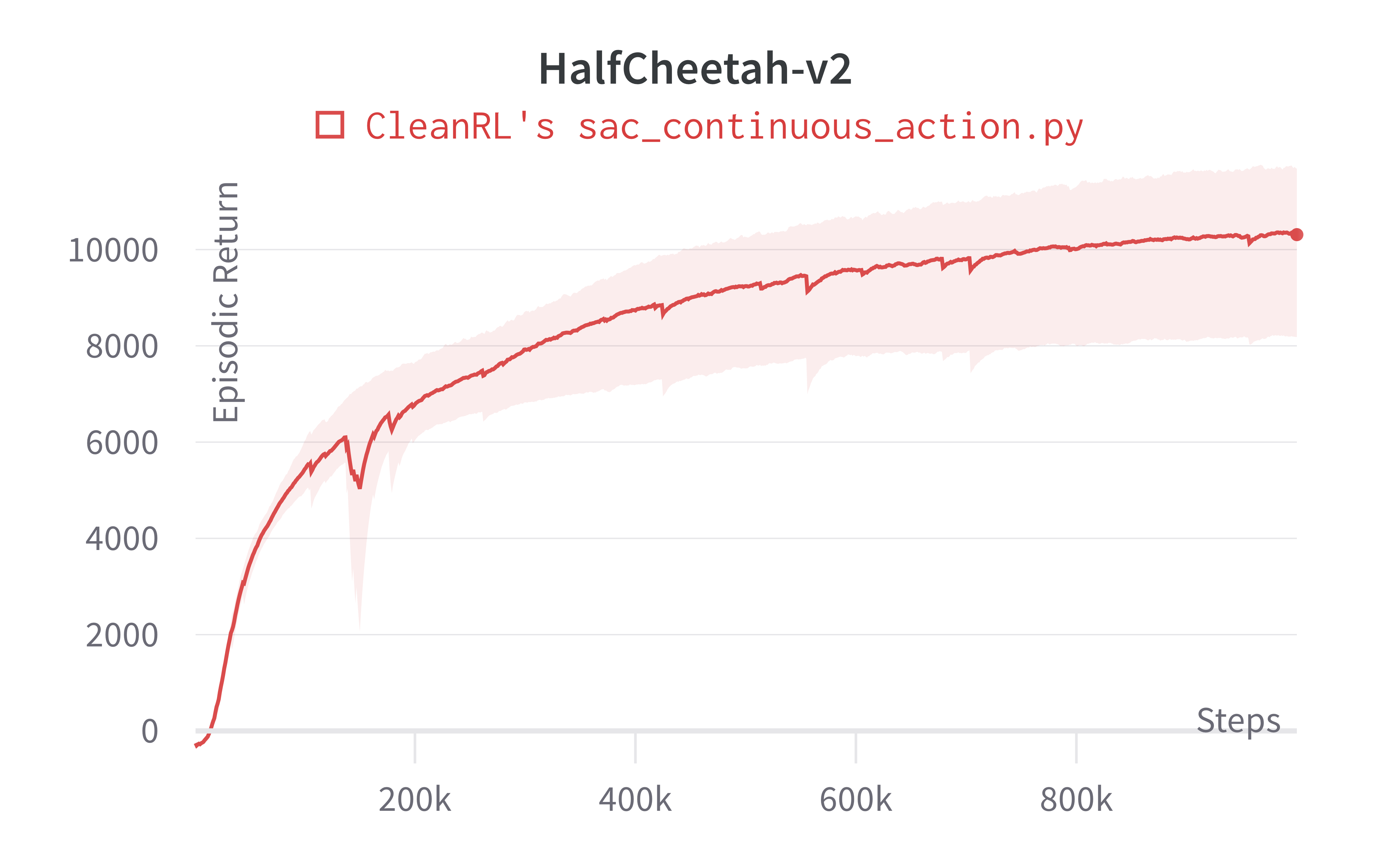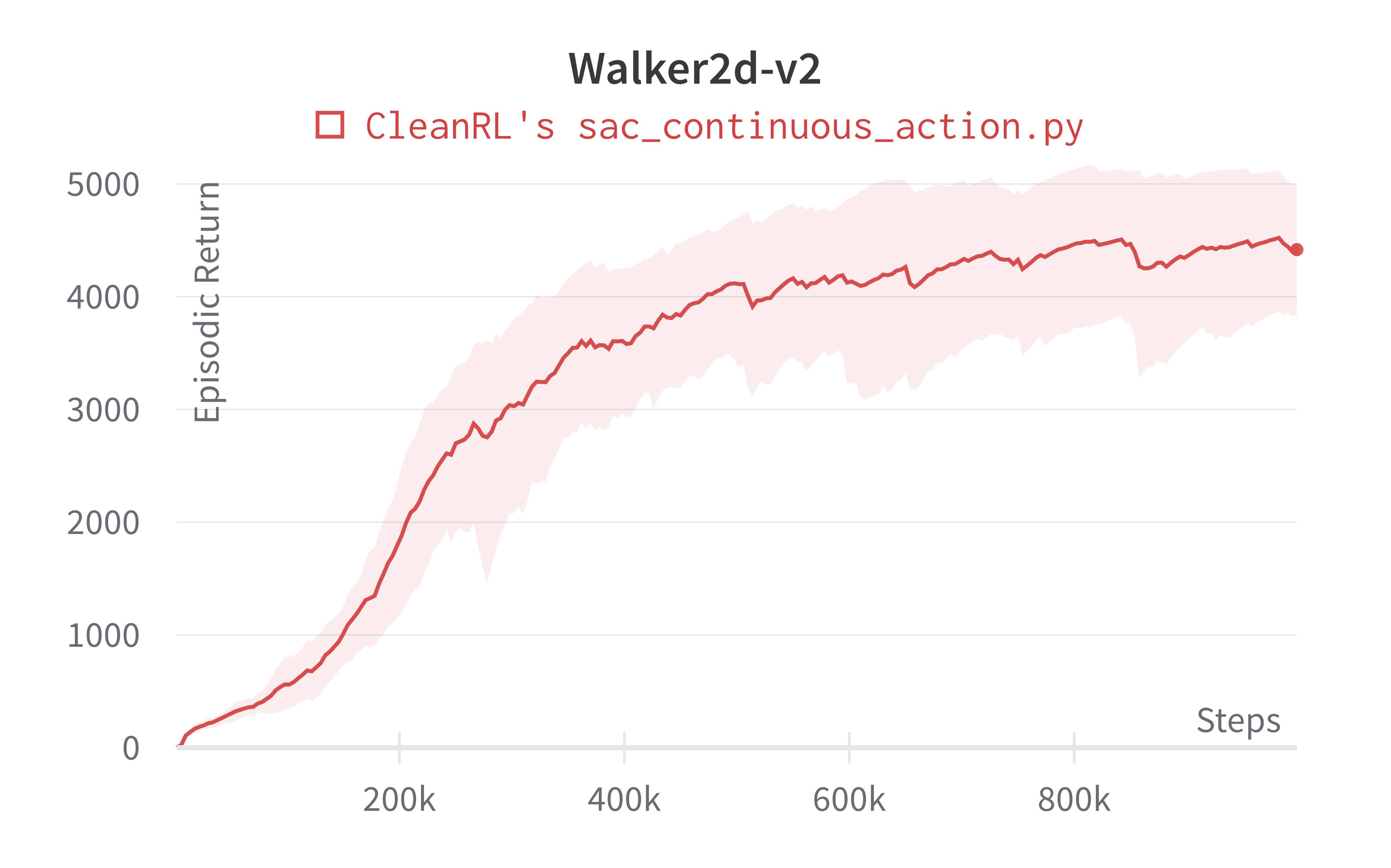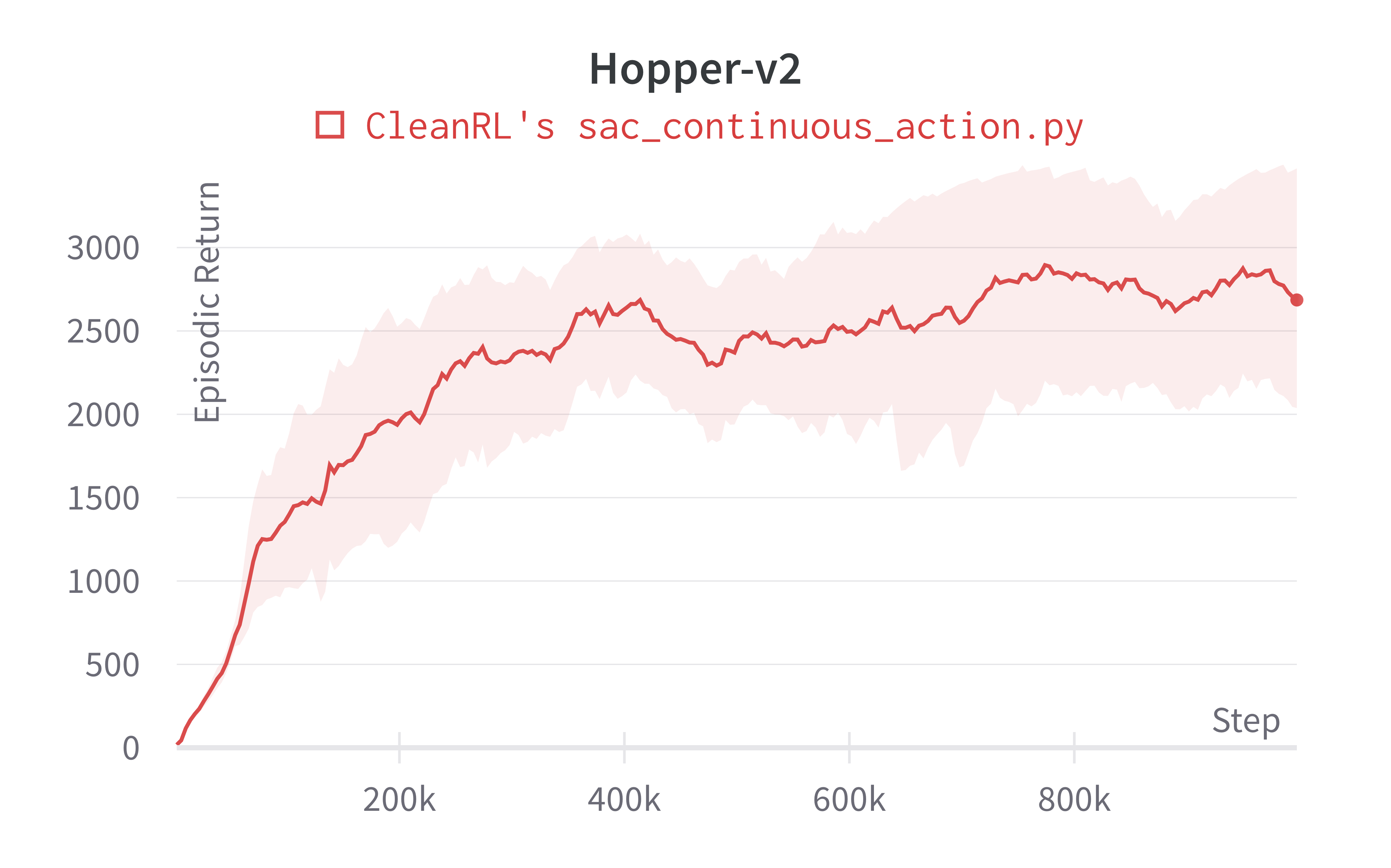Tracked experiments and game play videos:

## sac_atari.py

The sac_atari.py has the following features:

• For discrete action spaces.
• Works with the Box observation space of low-level features.
• Works with the Discrete action space.
• Improved stability and wall-clock efficiency through updates only every n-th step.
• Supports automatic entropy coefficient $$\alpha$$ tuning, enabled by default.

### Usage for discrete action spaces

poetry install
poetry install -E atari
poetry run python cleanrl/sac_atari.py.py --env-id PongNoFrameskip-v4
poetry run python cleanrl/sac_atari.py.py --env-id PongNoFrameskip-v4 --autotune False --alpha 0.2

pip install -r requirements/requirements-atari.txt
python cleanrl/sac_atari.py.py --env-id PongNoFrameskip-v4
python cleanrl/sac_atari.py.py --env-id PongNoFrameskip-v4 --autotune False --alpha 0.2


### Explanation of the logged metrics

The metrics logged by python cleanrl/sac_atari.py are the same as the ones logged by python cleanrl/sac_continuous_action.py (see the related docs). However, the computations for the objectives differ in some details highlighted below:

• losses/qf1_loss, losses/qf2_loss: for each Soft Q-value network $$Q_{\theta_i}$$, $$i \in \{1,2\}$$, this metric holds the mean squared error (MSE) between the soft Q-value estimate $$Q_{\theta_i}(s, a)$$ and the entropy regularized Bellman update target estimated as $$r_t + \gamma \, Q_{\theta_{i}^{'}}(s', a') + \alpha \, \mathcal{H} \big[ \pi(a' \vert s') \big]$$.

SAC-discrete is able to exploit the discrete action space by using the full action distribution to calculate the Soft Q-targets instead of relying on a Monte Carlo approximation from a single Q-value. The new Soft Q-target is stated below with differences to the continuous SAC target highlighted in orange: $$y = r(s, a) + \gamma \, {\color{orange}\pi (a | s^\prime)^{\mathsf T}} \Big(\min_{\theta_{1,2}} {\color{orange}Q_{\theta_i^{'}}(s')} - \alpha \, \log \pi( \cdot \vert s')\Big)~,$$

Note how in the discrete setting the Q-function $$Q_{\theta_i^{'}}(s')$$ is a mapping $$Q:S \rightarrow \mathbb R^{|\mathcal A|}$$ that only takes states as inputs and outputs Q-values for all actions. Using all this available information and additionally weighing the target by the corresponding action selection probability reduces variance of the gradient.

• losses/actor_loss: Given the stochastic nature of the policy in SAC, the actor (or policy) objective is formulated so as to maximize the likelihood of actions $$a \sim \pi( \cdot \vert s)$$ that would result in high Q-value estimate $$Q(s, a)$$. Additionally, the policy objective encourages the policy to maintain its entropy high enough to help explore, discover, and capture multi-modal optimal policies.

SAC-discrete uses an action probability-weighted (highlighted in orange) objective given as:

$\text{max}_{\phi} \, J_{\pi}(\phi) = \mathbb{E}_{s \sim \mathcal{D}} \bigg[ {\color{orange}\pi (a | s)^{\mathsf T}} \Big( \text{min}_{i=1,2} {\color{orange} Q_{\theta_i}(s)} - \alpha \, \log\pi_{\phi}(a \vert s) \Big) \bigg]$

Unlike for continuous action spaces, there is no need for the reparameterization trick due to using a Categorical policy. Similar to the critic objective, the Q-function $$Q_{\theta_i}(s)$$ is a function from states to real numbers and does not require actions as inputs.

• losses/alpha_loss: In the policy's objective defined above, the coefficient of the entropy bonus $$\alpha$$ is kept fixed all across the training. As suggested by the authors in Section 5 of the Soft Actor-Critic And Applications paper, the original purpose of augmenting the standard reward with the entropy of the policy is to encourage exploration of not well enough explored states (thus high entropy). Conversely, for states where the policy has already learned a near-optimal policy, it would be preferable to reduce the entropy bonus of the policy, so that it does not become sub-optimal due to the entropy maximization incentive.

In SAC-discrete, it is possible to weigh the target for the entropy coefficient by the policy's action selection probabilities to reduce gradient variance. This is the same trick that was already used in the critic and actor objectives, with differences to the regular SAC objective marked in orange:

$\alpha^{*}_t = \text{argmin}_{\alpha_t} \mathbb{E}_{a_t \sim \pi^{*}_t} \, {\color{orange}\pi (a | s)^{\mathsf T}}\big[ -\alpha_t \, \log\pi^{*}_t(a_t \vert s_t; \alpha_t) - \alpha_t \tau \mathcal{H} \big],$

Since SAC-discrete uses a Categorical policy in a discrete action space, a different entropy target is required. The author uses the maximum entropy Categorical distribution (assigning uniform probability to all available actions) scaled by a factor of $$\tau~.$$

### Implementation details

sac_atari.py uses the wrappers highlighted in the "9 Atari implementation details" in The 37 Implementation Details of Proximal Policy Optimization, which are as follows:

1. The Use of NoopResetEnv ( common/atari_wrappers.py#L12)
2. The Use of MaxAndSkipEnv ( common/atari_wrappers.py#L97)
3. The Use of EpisodicLifeEnv ( common/atari_wrappers.py#L61)
4. The Use of FireResetEnv ( common/atari_wrappers.py#L41)
5. The Use of WarpFrame (Image transformation) common/atari_wrappers.py#L134
6. The Use of ClipRewardEnv ( common/atari_wrappers.py#L125)
7. The Use of FrameStack ( common/atari_wrappers.py#L188)
8. Shared Nature-CNN network for the policy and value functions ( common/policies.py#L157, common/models.py#L15-L26)
9. Scaling the Images to Range [0, 1] ( common/models.py#L19)

Other noteworthy implementation details apart from the Atari wrapping are as follows:

1. sac_atari.py initializes the weights of its networks using He initialization (named after its author Kaiming He) from the paper "Delving Deep into Rectifiers: Surpassing Human-Level Performance on ImageNet Classification". The corresponding function in PyTorch is kaiming_normal_, its documentation can be found here. In essence, it means the weights of each layer are initialized according to a Normal distribution with mean $$\mu=0$$ and

$\sigma = \frac{\text{gain}}{\sqrt{\text{fan}}}~,$

where $$\text{fan}$$ is the number of input neurons to the layer and $$\text{gain}$$ is a constant set to $$\sqrt{2}$$ for ReLU layers.

2. sac_atari.py uses the Adam2 optimizer with an increased $$\epsilon$$-parameter to improve its stability. This results in an increase in the denominator of the update rule:

$\frac{\hat{m}_t}{\sqrt{\hat{v}_t} + \epsilon}$

Here $$\hat{m}_t$$ is the bias-corrected first moment and $$\hat{v}_t$$ the bias-corrected second raw moment.

3. sac_atari.py uses the action selection probabilities of the policy in multiple places to reduce the variance of gradient estimates. The target for the Soft Q-value estimate is weighted accordingly:

# CRITIC training
_, next_state_log_pi, next_state_action_probs = actor.get_action(data.next_observations)
qf1_next_target = qf1_target(data.next_observations)
qf2_next_target = qf2_target(data.next_observations)
# we can use the action probabilities instead of MC sampling to estimate the expectation
min_qf_next_target = next_state_action_probs * (
torch.min(qf1_next_target, qf2_next_target) - alpha * next_state_log_pi
)


A similar action-probability weighting can be used for the actor gradient:

_, log_pi, action_probs = actor.get_action(data.observations)
qf1_values = qf1(data.observations)
qf2_values = qf2(data.observations)
min_qf_values = torch.min(qf1_values, qf2_values)
# no need for reparameterization, the expectation can be calculated for discrete actions
actor_loss = (action_probs * ((alpha * log_pi) - min_qf_values)).mean()


Lastly, this variance reduction scheme is also used when automatic entropy tuning is enabled:

if args.autotune:
# re-use action probabilities for temperature loss
alpha_loss = (action_probs.detach() * (-log_alpha.exp() * (log_pi + target_entropy).detach())).mean()

alpha_loss.backward()
a_optimizer.step()
alpha = log_alpha.exp().item()

4. sac_atari.py uses --target-entropy-scale=0.89 while the SAC-discrete paper uses --target-entropy-scale=0.98 due to improved stability when training for more than 100k steps. Tuning this parameter to the environment at hand is advised and can lead to significant performance gains.

5. sac_atari.py performs learning updates only on every $$n^{\text{th}}$$ step. This leads to improved stability and prevents the agent's performance from degenerating during longer training runs.
Note the difference to sac_continuous_action.py: sac_atari.py updates every $$n^{\text{th}}$$ environment step and does a single update of actor and critic on every update step. sac_continuous_action.py updates the critic every step and the actor every $$n^{\text{th}}$$ step. It then compensates for the delayed actor updates by performing $$n$$ actor update steps.

6. sac_atari.py handles truncation and termination properly like (Mnih et al., 2015)3 by using SB3's replay buffer's handle_timeout_termination=True.

### Atari experiment results for SAC-discrete

Run benchmarks for benchmark/sac_atari.sh by executing:

The table below compares the results of CleanRL's sac_atari.py with the original paper results.

Info

Note that the results table above references the training episodic return for sac_atari.py without evaluation mode.

Environment sac_atari.py 100k steps SAC for Discrete Action Settings 100k steps sac_atari.py 5M steps dqn_atari.py 10M steps
PongNoFrameskip-v4 ~ -20.21 ± 0.62 -20.98 ± 0.0 ~19.24 ± 1.81 20.25 ± 0.41
BreakoutNoFrameskip-v4 ~2.33 ± 1.28 - ~343.66 ± 93.34 366.928 ± 39.89
BeamRiderNoFrameskip-v4 ~396.15 ± 155.81 432.1 ± 44.0 ~8658.97 ± 1554.66 6673.24 ± 1434.37

Learning curves: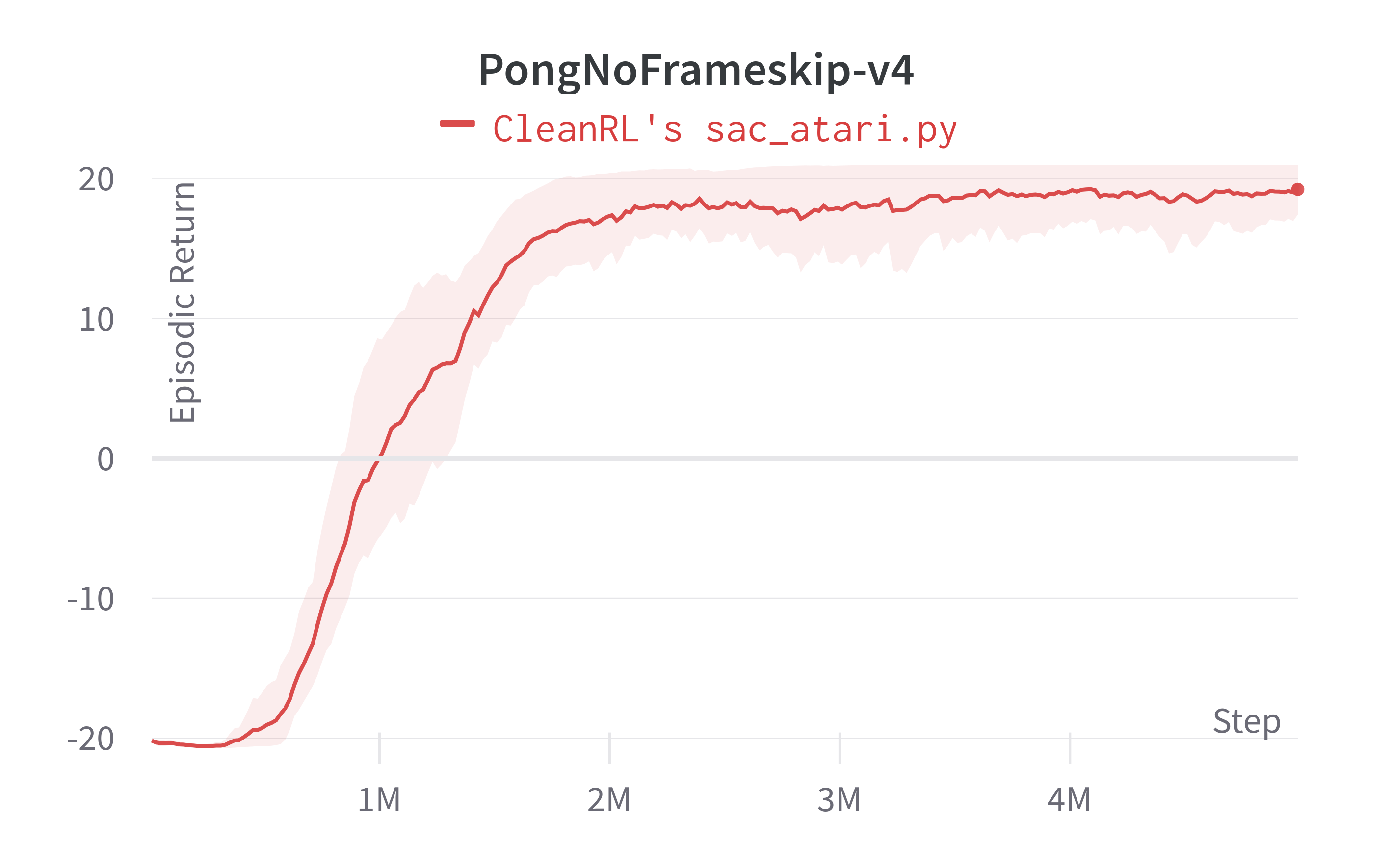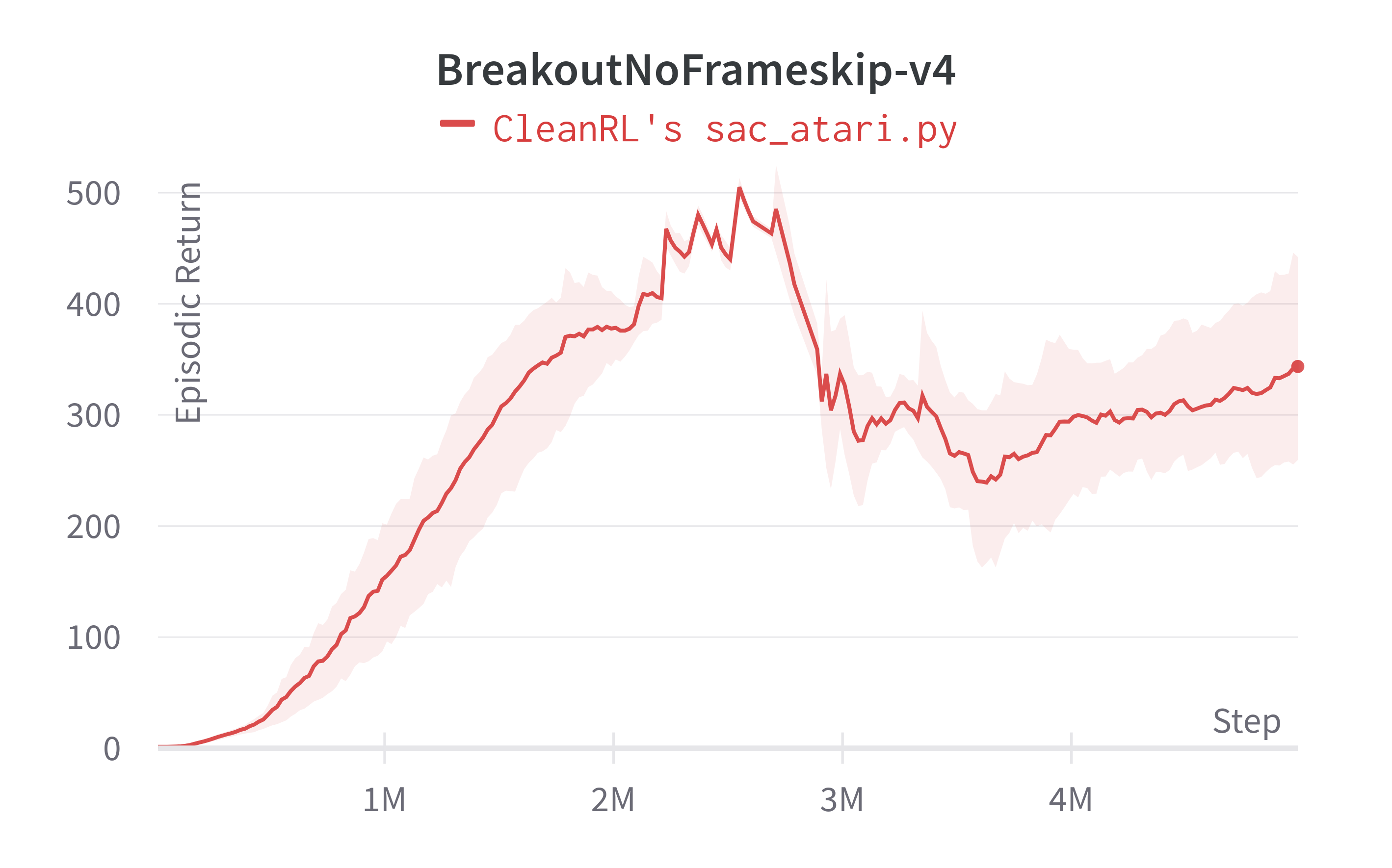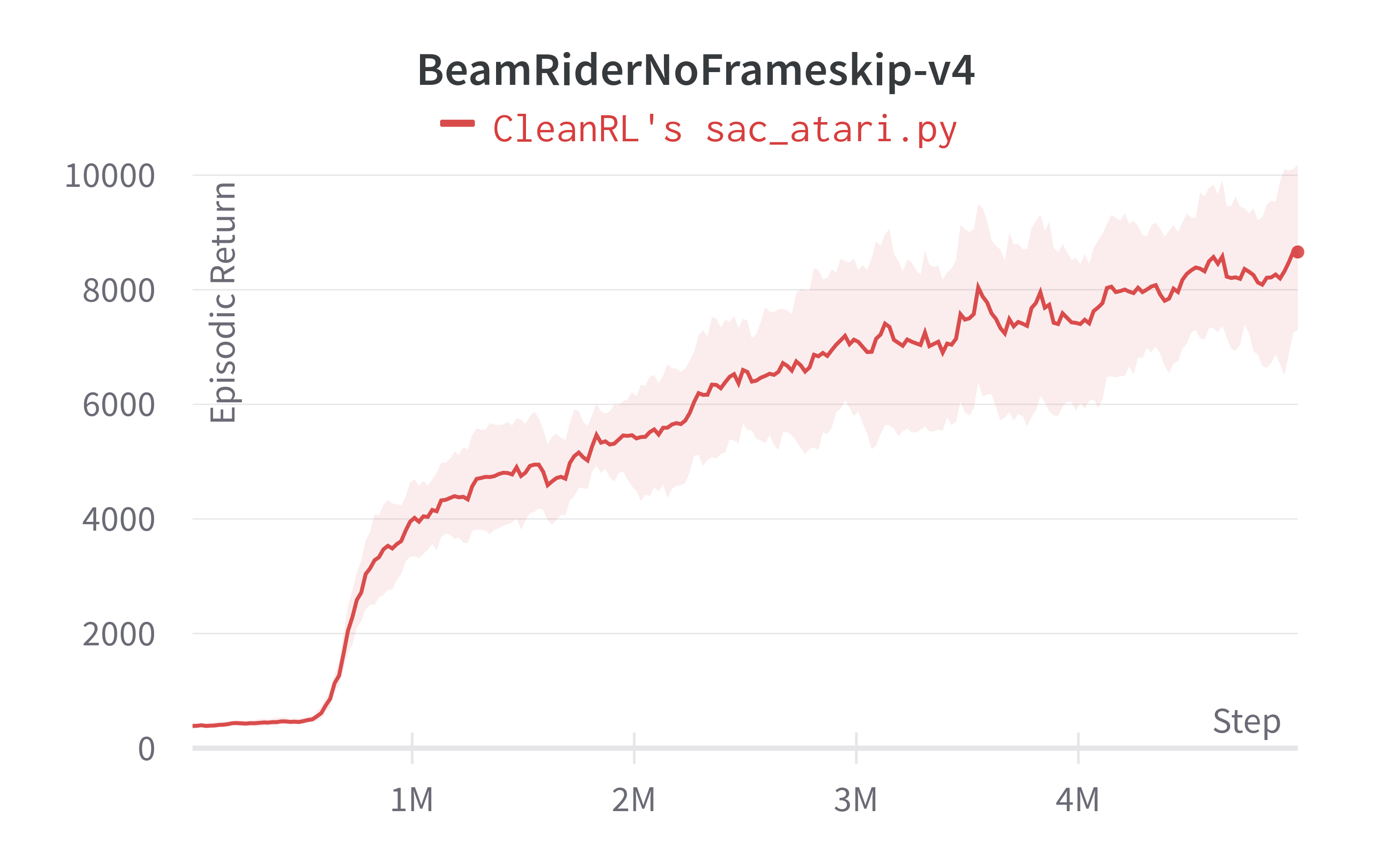Tracked experiments:

1. Diederik P Kingma, Max Welling (2016). Auto-Encoding Variational Bayes. ArXiv, abs/1312.6114. https://arxiv.org/abs/1312.6114

2. Diederik P Kingma, Jimmy Lei Ba (2015). Adam: A Method for Stochastic Optimization. ArXiv, abs/1412.6980. https://arxiv.org/abs/1412.6980

3. Mnih, V., Kavukcuoglu, K., Silver, D. et al. Human-level control through deep reinforcement learning. Nature 518, 529–533 (2015). https://doi.org/10.1038/nature14236